Heaviest & Lightest
Word Problems
Mental Math Subtraction
Fact Families
100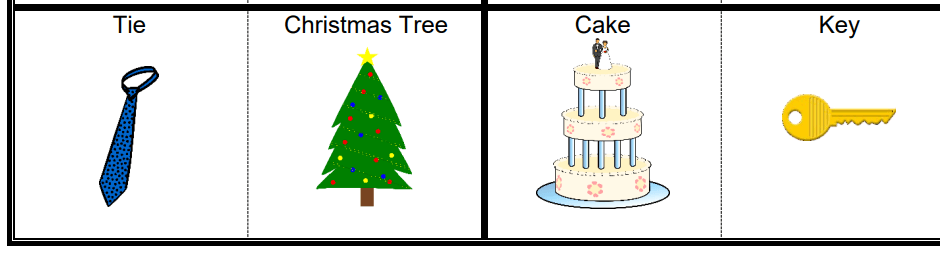The Christmas tree is the heaviest and the tie is the lightest.

100

Hunter, Adam and Peter went to the zoo. Hunter saw 15  penguins, Adam saw 8 penguins and Peter saw 6 penguins. How many penguins did the see in all?

15 + 8 + 6 = 29

100

19 + 2 = ?

19 + 2 = 21

100

18 - 16 = ?

18 - 16 = 2

100

? + 4 = 15

11 + 4 = 15

200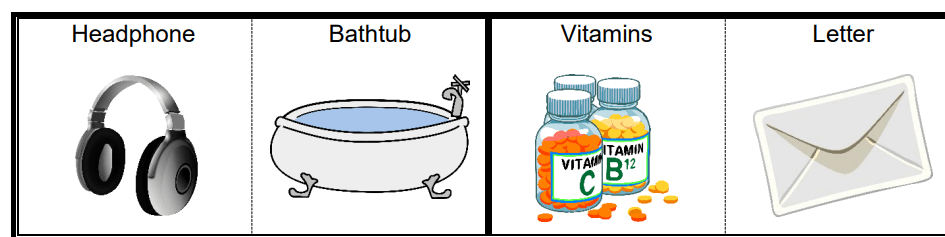The bathtub is the heaviest and the letter is the lightest.

200

Ava, Anne and Anna all swim. Last week Ava swam 3 hours, Anne swam 5 hours and Anna swam 2 hours. How many more hours did Anne swim than Ave?

5 -  3 = 2 Anne swam 2 hour more than Ava.

200

11 + 18 = ?

11 + 18 = 29

200

16 - 9 = ?

16 - 9 = 7

200

12 + ? = 23

12 + 11 = 23

300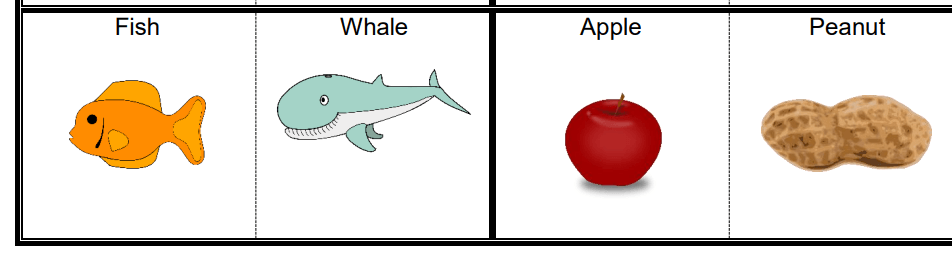The whale is the heaviest and the peanut is the lightest.

300

Last year Rose hit 16 home runs and Megan hit 3 home runs. How many more did Megan hit than Rose?

16 - 3 = 13

300

22 + 17= ?

22 + 17 = 39

300

31 - 21 = ?

31 - 21 = 10

300

19 - ? = 2

19 - 17 = 2

400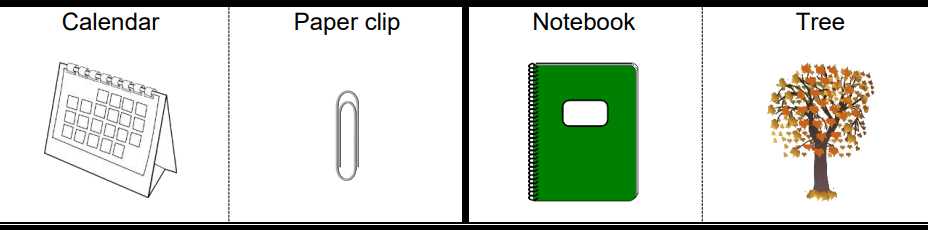the tree is the heaviest and the paper clip is the lightest.

400

Cindy is 6 years older than her brother. He is 14 years old. How old is Cindy?

14 = 6 = 20 Cindy is 20 years old.

400

7 + 11 = ?

18
400

91 - 72 = ?

91 - 72 = 19

400

30 - ? = 16

30 - 14 = 16

500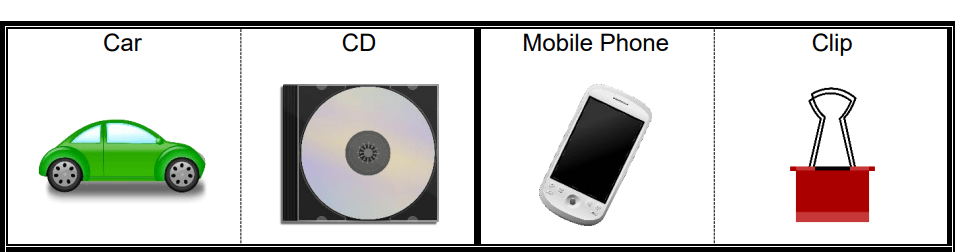The car is the heaviest and the paper clip is the lightest.

500

Emily and Emma helped clean up the park. Emma picked up 6 more bags of trash than Emily. Emma picked up 13 bags of trash How many bags of trash did Emily pick up?

13 - 6 = 7

500

21 + 28 = ?

21 + 28 = 49

500

86 - 19 = ?

86 - 19 = 67

500

28 - ? = 12

28 - 16 = 12
Click to zoom